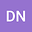An introduction to analysis of R\’enyi complexity ratio of quantum states for central potential
•## Abstract

R\’enyi complexity ratio of two density functions is introduced for three and multidimensional quantum systems. Localization property of several density functions are defined and five theorems about near continuous property of R\’enyi complexity ratio are proved by Lebesgue measure. Some properties of R\’enyi complexity ratio are demonstrated and investigated for different quantum systems. Exact analytical forms of R\’enyi entropy, R\’enyi complexity ratio, statistical complexities based on R\’enyi entropy for integral order have been presented for solutions of pseudoharmonic and a family of isospectral potentials. Some properties of R\’enyi complexity ratio are verified for six diatomic molecules (CO, NO, N$_2$, CH, H$_2$, and ScH) and for other quantum systems.

#### Peer review status:ACCEPTED

29 Jun 2021Submitted to International Journal of Quantum Chemistry
30 Jun 2021Submission Checks Completed
30 Jun 2021Assigned to Editor
14 Jul 2021Reviewer(s) Assigned
06 Aug 2021Review(s) Completed, Editorial Evaluation Pending
09 Aug 2021Editorial Decision: Revise Minor